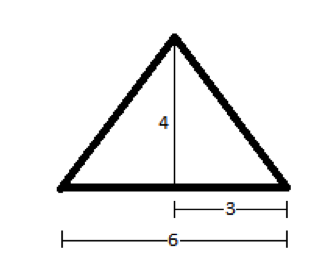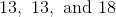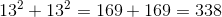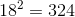# SAT Math : How to find the length of the side of an acute / obtuse isosceles triangle

## Example Questions

### Example Question #31 : Acute / Obtuse Triangles

An isosceles triangle has an area of 12. If the ratio of the base to the height is 3:2, what is the length of the two equal sides?

5

4

6

4√3

3√3

5

Explanation:

Area of a triangle is ½ x base x height. Since base:height = 3:2, base = 1.5 height.  Area = 12 = ½ x 1.5 height x height or 24/1.5 = height2.  Height = 4.  Base = 1.5 height = 6. Half the base and the height form the legs of a right triangle, with an equal leg of the isosceles triangle as the hypotenuse. This is a 3-4-5 right triangle.### Example Question #12 : Isosceles Triangles

Two sides of a triangle each have length 6. All of the following could be the length of the third side EXCEPT

12
2
3
11
1
Explanation:

This question is about the Triangle Inequality, which states that in a triangle with two sides A and B, the third side must be greater than the absolute value of the difference between A and B and smaller than the sum of A and B.

Applying the Triangle Inequality to this problem, we see that the third side must be greater than the absolute value of the difference between the other two sides, which is |6-6|=0, and smaller than the sum of the two other sides, which is 6+6=12. The only answer choice that does not satisfy this range of possible values is 12 since the third side must be LESS than 12.

### Example Question #13 : Isosceles Triangles

A triangle has the following side lengths:Which of the following correctly describes the triangle?

Obtuse and scalene

Obtuse and isosceles

Acute and scalene

None of these

Acute and isosceles

Acute and isosceles

Explanation:

The triangle has two sides of equal length, 13, so it is by definition isosceles.

To determine whether the triangle is acute, right, or obtuse, compare the sum of the squares of the lengths of the two shortest sides to the square of the length of the longest side. The former quantity is equal toThe latter quantity is equal toThe former is greater than the latter; consequently, the triangle is acute. The correct response is that the triangle is acute and isosceles.